# MP Board Class 7th Maths Solutions Chapter 8 Comparing Quantities Ex 8.2

## MP Board Class 7th Maths Solutions Chapter 8 Comparing Quantities Ex 8.2

Question 1.
Convert the given fractional numbers to percents.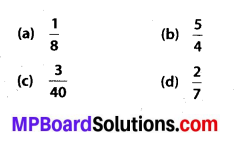Solution: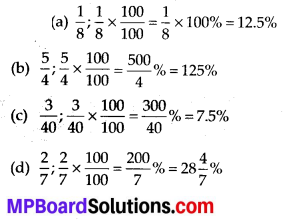Question 2.
Convert the given decimal fractions to percents.
(a) 0.65
(b) 2.1
(c) 0.02
(d) 12.35
Solution: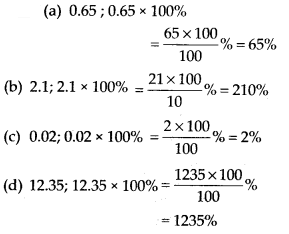Question 3.
Estimate what part of the figures is coloured and hence find the percent which is coloured.Solution:
(i) Here, 1 part out of 4 equals parts is shaded which represents the fraction $$\frac{1}{4}$$.(ii) Here, 3 parts out of 5 equal parts are shaded which represents the fraction $$\frac{3}{5}$$.(iii) Here 3 parts out of 8 equal parts are shaded which represents the fraction $$\frac{3}{8}$$.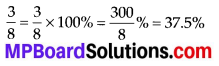Question 4.
Find:
(a) 15% of 250
(b) 1% of 1 hour
(c) 20% of ₹ 2500
(d) 75% of 1 kg
Solution: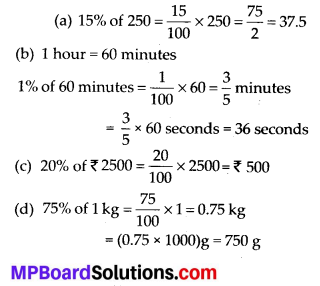Question 5.
Find the whole quantity if
(a) 5% of it is 600
(b) 12% of it is? 1080
(c) 40% of it is 500 km
(d) 70% of it is 14 minutes
(e) 8% of it is 40 litres
Solution:
Let the whole quantity be x.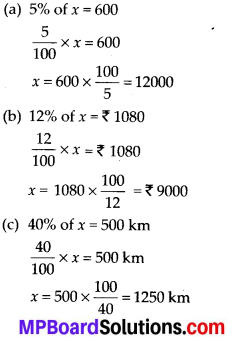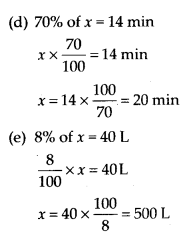Question 6.
Convert given percents to decimal fractions and also to fractions in simplest forms:
(a) 25%
(b) 150%
(c) 20%
(d) 5%
Solution: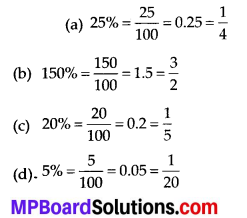Question 7.
In a city, 30% are females, 40% are males and remaining are children. What percent are children?
Solution:
It is given that 30% are females and 40% are males.
Children = 100% – (40% + 30%)
= 100% – 70% = 30%Question 8.
Out of 15,000 voters in a constituency, 60% voted. Find the percentage of voters who did not vote. Can you now find how many actually did not vote?
Solution:
Percentage of voters who voted = 60%
Percentage of those who did not vote = 100% – 60%
= 40%
Number of people who did not vote = 40% of 15000
= 40% × 15000Therefore, 6000 people did not vote.

Question 9.
Meeta saves ₹ 400 from her salary. If this is 10% of her salary. What is her salary?
Solution:
Let Meeta’s salary be ₹ x.
Given that, 10% of x = 400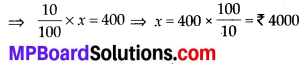Therefore, Meeta’s salary is ₹ 4000.

Question 10.
A local cricket team played 20 matches in one season. It won 25% of them. How many matches did they win?
Solution:
Number of games won = 25% of 20
$$=\frac{25}{100} \times 20=5$$
Therefore, the team won 5 matches.